#### Guitar Amp Design - A New Approach!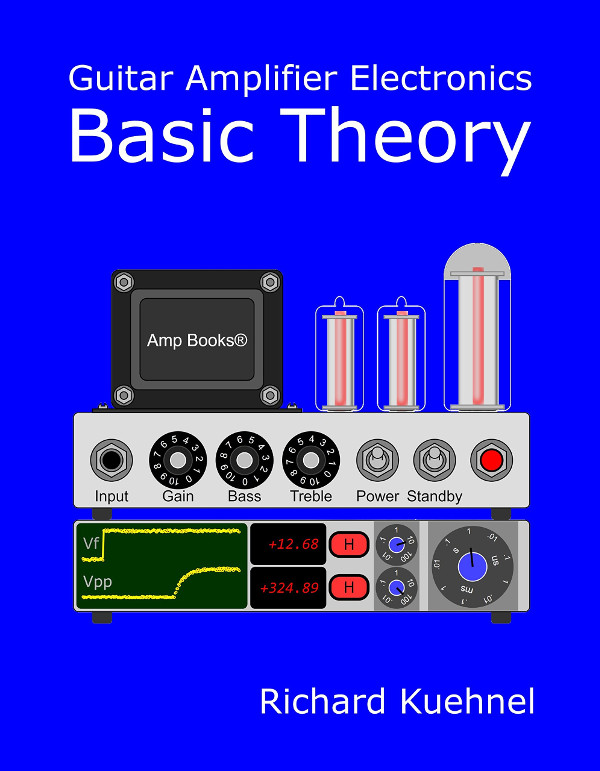Basic Theory - using 21st-Century technology to design vacuum tube circuitsSystem Design - a professional methodology for crafting a complete guitar amplifierCircuit Simulation - modeling vacuum tube circuits using SPICE

What water is to plumbing, electrons are to a vacuum tube circuit. The outer electrons of copper atoms, for example, flow freely from atom to atom in a wire. Each electron has a negative charge, and when we get a bunch of them to flow along a wire in one direction, we get a flow of charge called current. Charge is measured in units of coulombs.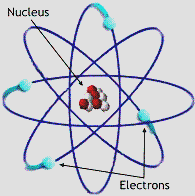It takes an enormous number of electrons to equal one coulomb. In fact, it takes about 6.2x1018. Looking at this from the opposite perspective, one electron has a negative charge equal to -1.6x10-19 coulombs.

One coulomb of charge flowing per second equals one ampere of current. Actually saying the word "ampere," however, makes me feel like an impressionist painter writing a line of iambic verse to describe dew-covered foliage on a cool spring morning. If you want to be an authentic tube-head then you need to say "amp." One thousandth of an ampere is a "milliamp."

One coulomb per second is equal to one amp. Example: I'm writing this from Germany where my EL34 push-pull amplifier draws about one ampere of current from the wall socket. So my amp draws an amp.

It takes a flow of 6.2x1018 electrons per second to produce one amp of current. Fortunately good conductors have a lot of electrons available for this task. In copper metal, for example, there are 8.5x1028 free electrons available in one cubic meter. OK, maybe we don't use a whole cubic meter of copper in a tube amp, but even smaller chunks have more than enough free electrons to meet our needs.

In vacuum tubes, electrons flow from the heated cathode to the unheated plate, so you would think that current would flow in that direction. This is, after all, the direction in which physical particles are actually moving. Alas, this is not the case. Electrons are negatively charged, and in terms of current, negatively charged particles moving in one direction have the same effect as positive charges flowing in the opposite direction. Thus we say that "positive" current flows from the plate to the cathode. It's a strange concept at first, but easy to get used to. We just need to imagine that the current is caused by positively charged particles, each with a charge of +1.6x10-19C, flowing in a direction opposite to the electron flow.

#### Problem

A 1cm length of 20AWG wire connects two terminals of the "speed" control of your Fender Tremolux 6G9. Its copper metal conductor contains 4.4x1020 free electrons capable of conducting current. How much charge do these free electrons represent?

#### Solution

The total charge is equal to the number of electrons times the charge per electron:

(4.4x1020)(-1.6x10-19) = -70C

Not shabby for a tiny length of wire!

#### Problem

Through incredibly astute observation you notice that 1.25x1016 electrons flow each second from the cathode to the plate of your 12AX7 preamp. How many amps of current does this represent? In which direction?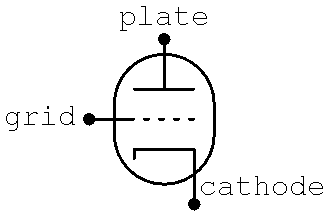#### Solution

1.25x1016 electrons represent a charge of

(1.25x1016)(-1.6x10-19) = -0.002C

This means -0.002C per second flows from the cathode to the plate. In terms of current, this is the same as if there were +0.002C per second flowing from the plate to the cathode. This equals 0.002A, or 2mA, where "A" is amps and "mA" is milliamps. Thus we have 2mA of current flowing from the plate to the cathode.

#### Problem

4.1x1018 electrons per minute travel from the power amp screen supply to the power amp plate supply via a choke. (I'm sure you had fun counting them!) What is the current in milliamps?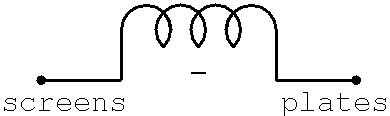#### Solution

If we divide by 60 then 4.1x1018 electrons per minute is equal to 6.8x1016 electrons per second. This means that the charge flowing through the choke, measured in coulombs per second, is

(6.8x1016C/s)(-1.6x10-19) = -0.011C/s

As far as current is concerned, this is the same as positive charges flowing in the opposite direction at a rate of +0.011C/s, which is 11mA. Thus 11mA of current flows from the plate supply through the choke to the screen supply. The electrons that make all this possible are actually flowing in the opposite direction, but for circuit analysis we don't care about this trivial detail.

This problem raises a big beef I have with physics. When it comes to describing charge, isn't plus or minus relative? Since we seem to use electrons for almost everything in electronics wouldn't it make our lives simpler to define electrons as being positive and the nucleus of the atom to be negative? We would then have positive charges flowing and not need to explain the difference between the fantasy of our circuit designs and physical reality.

This could be interesting... In a positive-electron world we would use negative power supplies to make our 12AX7 plates highly negative. This would attract the positive electrons and make positive current flow from the cathode to the plate, the same direction as the positive charges. The grid would be biased to a positive voltage to suppress electron flow. After all, positive repels positive. When it comes to electrons we would simply need to think positive thoughts. Works for me. OK back to reality.

#### Problem

Your Champ 5E1 clone uses 20AWG wire with a diameter of 0.812mm to connect the plate supply voltage to the 100k plate resistor. The resistor carries a current of 0.6mA at idle with no guitar signal. How fast are the electrons flowing through the wire? Could you use a larger diameter wire to make them flow faster?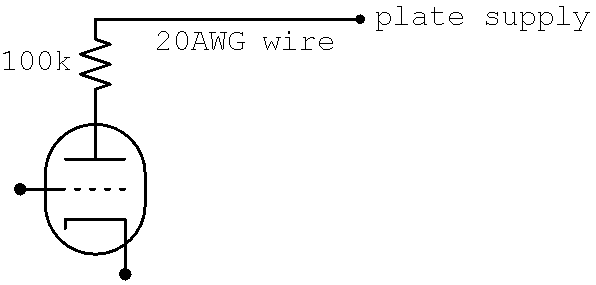#### Solution

The radius of the wire is half the diameter, or 0.406mm, which is 4.06x10-4 meters. We get the area by squaring the radius and multiplying by pi. This means a cross section has an area of

(3.14)(4.06x10-4)2 = 5.2x10-7

square meters. There are 8.5x1028 free electrons in one cubic meter of copper. This means that in one meter of 20AWG wire there are

(8.5x1028)(5.2x10-7) = 4.4x1022

electrons. To get the amount of charge in one meter of wire we multiply by the number of coulombs per electron, giving us

(4.4x1022)(-1.6x10-19) = -7.0x103C

Looking at this from the opposite perspective, this means it takes

1/(7.0x103) = 1.4x10-4

meters of wire to contain -1C of charge. This is less than a millimeter! And we're talking about a fairly thin wire. We know that the current through the plate circuit is 0.6mA, which means 6x10-4C of charge flows through the wire each second. (This is positive current from the plate supply through the plate resistor to the plate of the tube, as if there were positive charges moving through the wire.)

The number of meters of wire containing -0.0006C of charge is

(1.4x10-4)(6x10-4) = 8x10-8

So this number of meters worth of charge that flows through the wire each second. Thus the electrons are drifting through the wire at a speed of 8x10-8 meters per second. If you used a larger diameter wire then there would be more electrons in a cross section. The electrons would thus travel more slowly to produce the same amount of current.

As this problem demonstrates, electrons drift very slowly. In this case only 7.4 millimeters in an entire day! It's important to keep in mind, however, that guitar signals travel nearly the speed of light. If we pump a current of 0.6mA into one end of the wire, then 0.6mA will flow out the other end almost instantaneously. Just don't expect to see the same electrons coming out that you put in.

You and thousands of other motorists are stopped on the Golden State Freeway between San Fernando and Burbank. If all of you would simply move forward at the same time, then the "signal" to move forward would propagate instantaneously. If you're in the left lane back in San Fernando, however, you still won't be seeing Exit 144 for a very long time.

#### Problem

At full power and maximum supply voltage sag, the Fender Bassman 5F6-A power supply sustains a load of 187mA. How many coulombs per second does this represent? The power supply under these conditions provides +377 volts DC to the load. In which direction through the load do the electrons flow?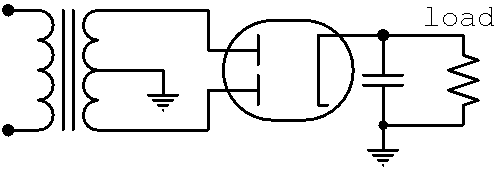Guitar Amplifier Electronics: Basic Theory - a 21st-Century approach to understanding preamp, power amp, and power supply designFundamentals of Guitar Amplifier System Design - a structured methodology for crafting an awesome guitar amplifier!Guitar Amplifier Electronics: Circuit Simulation - a deep dive into SPICE tube amp modeling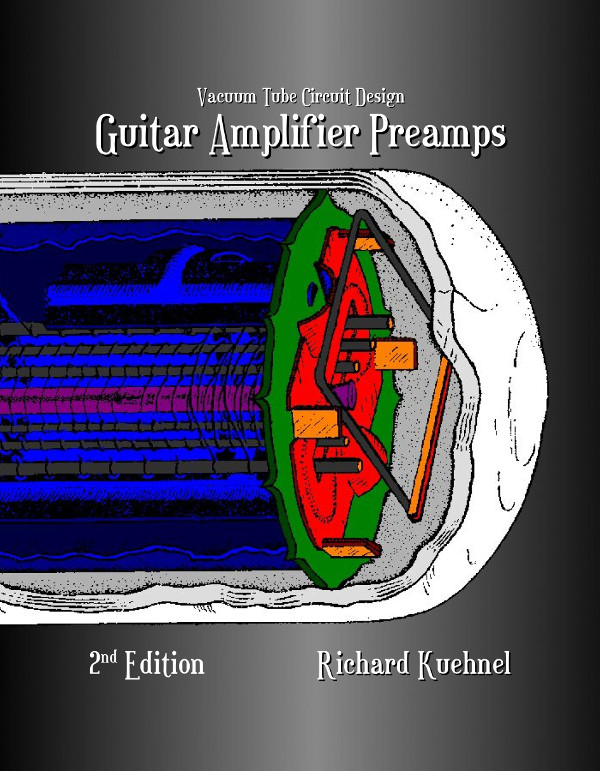Guitar Amplifier Preamps - upstream circuits using triodes and pentodes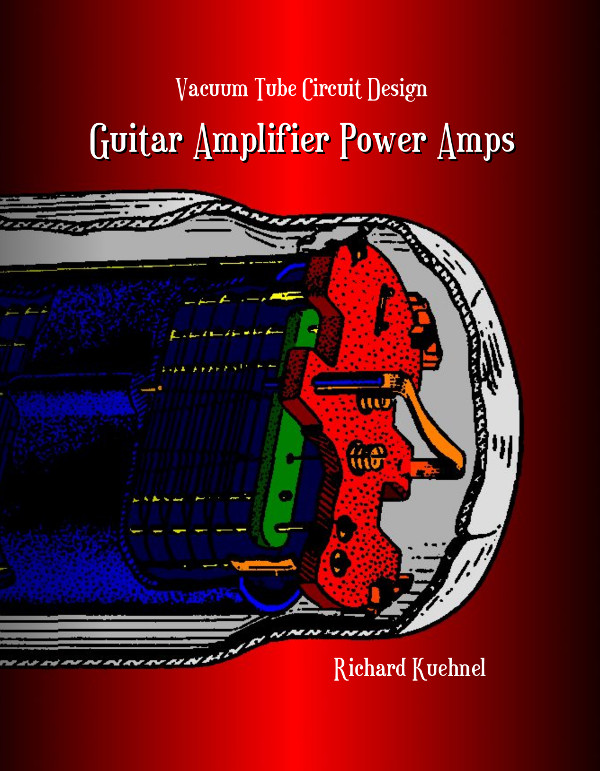Guitar Amplifier Power Amps - from the phase inverter to the loudspeakerThe Fender Bassman 5F6-A - plus Marshall's Bluesbreaker and Plexi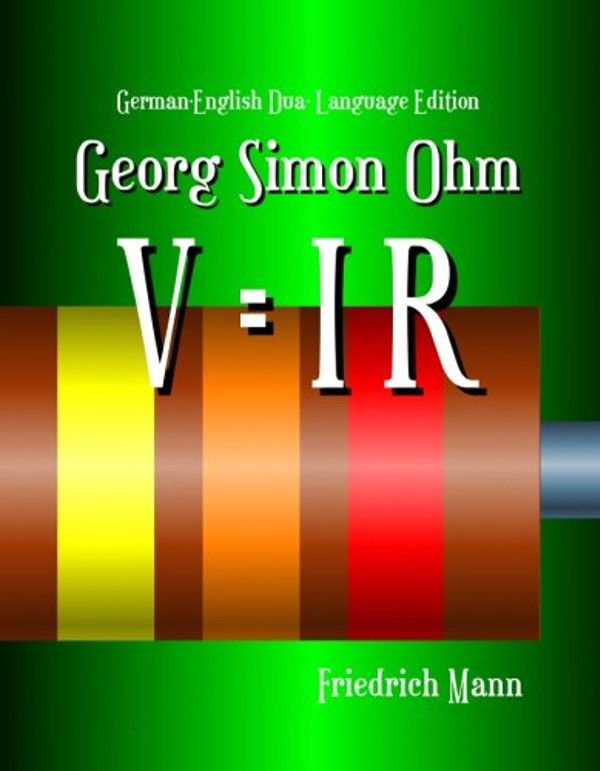Georg Simon Ohm - Imagine a world without Ohm's Law!